# MNE : From raw data to epochs and evoked responses (ERF/ERP)¶

Author : Alexandre Gramfort

## Introduction

This tutorial describes how to define epochs-of-interest (trials) from your recorded MEG-data, and how to apply the different preprocessing steps.

Subsequently, you will find information how to compute an event related potential (ERP)/ event related field (ERF) and how to handle the three channels that record the signal at each sensor location. It explains how to visualize the axial magnetometer and planar gradiometer signals.

## Background to preprocessing

In MNE the preprocessing of data refers to the reading of the data, segmenting the data around interesting events such as triggers, temporal filtering and optionally rereferencing.

MNE works by loading data in memory only if needed when extracting epochs. The raw data do not need to fit in memory unless you want to filter continuous data (see below). In the introduction, the filtering of continuous data is optional.

Preprocessing involves several steps including identifying individual trials (called Epochs in MNE) from the dataset (called Raw), filtering and rejection of bad epochs. This tutorial covers how to identify trials using the trigger signal. Defining data segments of interest can be done according to a specified trigger channel and the use of an array of events.

## Background to averaging

When analyzing EEG or MEG signals, the aim is to investigate the modulation of the measured brain signals with respect to a certain event. However, due to intrinsic and extrinsic noise in the signals - which in single trials is often higher than the signal evoked by the brain - it is typically required to average data from several trials to increase the signal-to-noise ratio(SNR). One approach is to repeat a given event in your experiment and average the corresponding EEG/MEG signals. The assumption is that the noise is independent of the events and thus reduced when averaging, while the effect of interest is time-locked to the event. The approach results in ERPs and ERFs for respectively EEG and MEG. Timelock analysis can be used to calculate ERPs/ ERFs.

## Dataset: MEG median nerve stimulation

The MEG dataset used in this tutorial are recorded during electrical stimulation of the Median Nerve (MN) of the left hand.

The MEG signals were recorded with the NatMEG 306 sensor Neuromag Triux system. The Neuromag MEG system has 306 channels located at 102 unique locations in the dewar. Of these, 102 channels are axial magnetometer sensors that measure the magnetic field in the radial direction, i.e. orthogonal to the scalp. The other 2*102 channels are planar gradiometers, which measure the magnetic field gradient tangential to the scalp. The planar gradient MEG data has the advantage that the amplitude typically is the largest directly above a source.

In :
# add plot inline in the page (not necessary in Spyder)
%matplotlib inline


First, load the mne package:

In :
import mne


We set the log-level to 'WARNING' so the output is less verbose

In :
mne.set_log_level('WARNING')


## Access raw data¶

In :
data_path = '/Users/alex/Sync/karolinska_teaching/'
raw_fname = data_path + '/meg/somstim_raw.fif'

print raw_fname

/Users/alex/Sync/karolinska_teaching//meg/somstim_raw.fif



Read data from file:

In :
raw = mne.fiff.Raw(raw_fname)
print raw

<Raw  |  n_channels x n_times : 324 x 826000>


In :
raw.ch_names[:20:3]

Out:
['BIO001', 'BIO004', 'IASY+', 'IASZ-', 'IAS_X', 'MEG0111', 'MEG0121']

In :
print raw.info

<Info | 24 non-empty fields
acq_pars : unicode | 25263 items
bads : list | 0 items
buffer_size_sec : numpy.float64 | 1.0
ch_names : list | BIO001, BIO002, BIO003, BIO004, IASX+, IASX-, IASY+, ...
chs : list | 324 items
comps : list | 0 items
description : unicode | 12 items
dev_head_t : dict | 3 items
dig : list | 117 items
experimenter : unicode | 15 items
file_id : dict | 4 items
filename : str | /Users/ale.../somstim_raw.fif
filenames : list | 1 items
highpass : float | 0.10000000149
lowpass : float | 330.0
meas_date : numpy.ndarray | 2014-01-13 15:02:54
meas_id : dict | 4 items
nchan : int | 324
orig_blocks : list | 5 items
orig_fid_str : instance | <StringIO.StringIO instance at 0x1105c3b00>
proj_id : numpy.ndarray | 1 items
proj_name : unicode | 8 items
projs : list | generated with autossp-1.0.1: off, ...
sfreq : float | 1000.0
acq_stim : NoneType
dev_ctf_t : NoneType
>



Look at the channels in raw:

In :
data, times = raw[:, :10]
print data.shape

(324, 10)



Read and plot a segment of raw data

In :
start, stop = raw.time_as_index([100, 115])  # 100 s to 115 s data segment
data, times = raw[:306, start:stop]
print data.shape
print times.shape
print times.min(), times.max()

(306, 15000)
(15000,)
100.0 114.999



Where are my magnetometers?

In :
picks = mne.fiff.pick_types(raw.info, meg='mag', exclude=[])
print picks

[ 15  18  21  24  27  30  33  36  39  42  45  48  51  54  57  60  63  66
69  72  75  78  81  84  87  90  93  96  99 102 105 108 111 114 117 120
123 126 129 132 135 138 141 144 147 150 153 156 159 162 165 168 171 174
177 180 183 186 189 192 195 198 201 204 207 210 213 216 219 222 225 228
231 234 237 240 243 246 249 252 255 258 261 264 267 270 273 276 279 282
285 288 291 294 297 300 303 306 309 312 315 318]



Take some magnetometer data and plot it

In :
picks = mne.fiff.pick_types(raw.info, meg='mag', exclude=[])
data, times = raw[picks[:10], start:stop]

import matplotlib.pyplot as plt
plt.plot(times, data.T)
plt.xlabel('time (s)')
plt.ylabel('MEG data (T)')

Out:
<matplotlib.text.Text at 0x1105d08d0>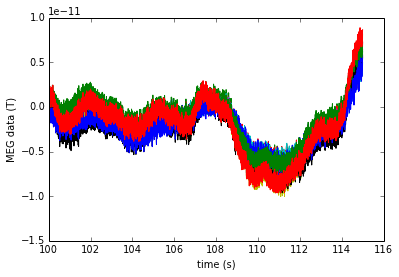In :
len(mne.fiff.pick_types(raw.info, meg='grad', eeg=False, exclude='bads'))

Out:
204

In :
raw.info['bads']  # there is no channel marked as bad here

Out:
[]


Save a segment of 150s of raw data (MEG only):

In :
picks = mne.fiff.pick_types(raw.info, meg=True, eeg=False, stim=True, exclude=[])
# raw.save('somsen_short_raw.fif', tmin=0., tmax=150., picks=picks, overwrite=True)


### Filtering (run only if you have enough memory and if you're patient):

Otherwise you can use mne_process_raw in the terminal with something like

mne_process_raw --raw somstim_raw.fif --highpass 1 --lowpass 40 --save somstim_raw_1_40_raw.fif --projoff --decim 4 --digtrig STI101

In :
# raw_beta = mne.fiff.Raw(raw_fname, preload=True)  # reload data with preload for filtering

# keep beta band
# raw_beta.filter(13.0, 30.0, method='iir')

# save the result
# raw_beta.save('somsen_beta_raw.fif', overwrite=True)

# print raw_beta.info


## Exercise :

• Filter the raw data between 1Hz and 40Hz. Observe the absence of the slow drifts.
• Plot the magnetometers and the gradiometers separately. Observe the different units / scales of the data.

## Define and read epochs¶

First extract events:

In :
events = mne.find_events(raw, stim_channel='STI101', verbose=True)

Reading 0 ... 825999  =      0.000 ...   825.999 secs...
[done]
400 events found
Events id: 


In :
print events[:5]  # events is a 2d array

[[23283     0     1]
[25342     0     1]
[27368     0     1]
[29386     0     1]
[31437     0     1]]


In :
len(events[events[:, 2] == 1])

Out:
400

In :
len(events)

Out:
400


Where are they coming from?

In :
d, t = raw[raw.ch_names.index('STI101'), :]
d.shape

Out:
(1, 826000)

In :
raw.ch_names.index('STI101')

Out:
321

In :
raw.ch_names[315:322]

Out:
['MEG2631', 'MEG2632', 'MEG2633', 'MEG2641', 'MEG2642', 'MEG2643', 'STI101']

In :
plt.plot(d[0,:100000])

Out:
[<matplotlib.lines.Line2D at 0x113d76150>]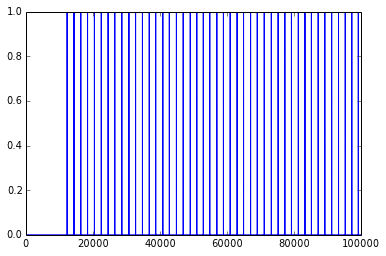Events are stored as 2D numpy array where the first column is the time instant and the last one is the event number. It is therefore easy to manipulate.

## Exercise

using the raw.info['sfreq'] attribute in the measurement info and the events array estimate the mean interstimulus interval

### !!! we need to fix now tiny acquisition problems

In :
def fix_info(raw):
raw.info['chs'][raw.ch_names.index('BIO001')]['kind'] = mne.fiff.constants.FIFF.FIFFV_EOG_CH
raw.info['chs'][raw.ch_names.index('BIO002')]['kind'] = mne.fiff.constants.FIFF.FIFFV_EOG_CH
raw.info['chs'][raw.ch_names.index('BIO003')]['kind'] = mne.fiff.constants.FIFF.FIFFV_ECG_CH
raw.info['chs'][raw.ch_names.index('BIO004')]['kind'] = mne.fiff.constants.FIFF.FIFFV_ECG_CH

fix_info(raw)

# check it worked
print mne.fiff.pick_types(raw.info, meg=False, eog=True, ecg=True)

[0 1 2 3]



# Now lets define our epochs and compute the ERF

We shall define epochs parameters. The first is set the event_id that is a Python dictionary to relate a condition name to the corresponding trigger number.

In :
event_id = dict(som=1)  # event trigger and conditions
print event_id

{'som': 1}



Then the pre and post stimulus time intervals. Times are always in seconds and relative to the stim onset.

In :
tmin = -0.1  # start of each epoch (200ms before the trigger)
tmax = 0.3  # end of each epoch (500ms after the trigger)


Mark some channels as bad (not necessary here):

In :
# raw.info['bads'] = ['MEG 2443']


The variable raw.info[‘bads’] is just a python list.

Pick the good channels:

In :
picks = mne.fiff.pick_types(raw.info, meg=True, eeg=True, eog=True,


Alternatively one can restrict to magnetometers or gradiometers with:

In :
mag_picks = mne.fiff.pick_types(raw.info, meg='mag', eog=True, exclude='bads')


Define the baseline period:

In :
baseline = (None, 0)  # means from the first instant to t = 0


Define peak-to-peak rejection parameters for gradiometers, magnetometers and EOG:

In :
reject = dict(grad=4000e-13, mag=4e-12, eog=150e-6)


In :
epochs = mne.Epochs(raw, events, event_id, tmin, tmax, proj=True,
picks=picks, baseline=baseline, reject=reject)
print epochs

<Epochs  |  n_events : 400 (good & bad), tmin : -0.1 (s), tmax : 0.3 (s), baseline : (None, 0)>



or if you want to preload

In :
epochs = mne.Epochs(raw, events, event_id, tmin, tmax, proj=True,
picks=picks, baseline=baseline, reject=reject,
preload=True)  # now data are in memory
print epochs

<Epochs  |  n_events : 367 (all good), tmin : -0.1 (s), tmax : 0.3 (s), baseline : (None, 0)>


In :
epochs.plot_drop_log()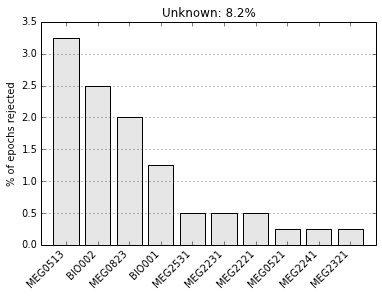Out:
8.25


Get the single epochs as a 3d array:

In :
epochs_data = epochs['som'].get_data()
print epochs_data.shape

(367, 308, 401)



epochs_data is a 3D array of dimension (367 epochs, 308 channels, 401 time instants).

Scipy supports reading and writing of matlab files. You can save your single trials with:

In :
# from scipy import io
# io.savemat('epochs_data.mat', dict(epochs_data=epochs_data), oned_as='row')


or if you want to keep all the information about the data you can save your epochs in a fif file:

In :
# epochs.save('somstim-epo.fif')


Compute evoked responses for somatosensory responses by averaging and plot it:

In :
evoked = epochs['som'].average()
print evoked

<Evoked  |  comment : 'som', time : [-0.100000, 0.300000], n_epochs : 367, n_channels x n_times : 306 x 401>


In :
evoked.save('somtim-ave.fif')  # save it to disk

In :
fig = evoked.plot()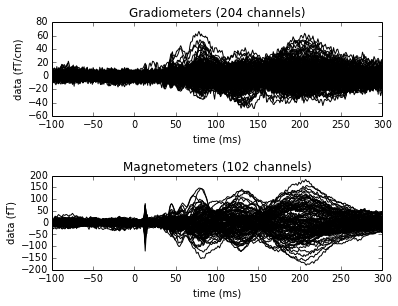In :
import numpy as np

# Ugly hack due to acquisition problem when specifying the channel types
layout.names = mne.utils._clean_names(layout.names, remove_whitespace=True)

fig = evoked.plot_topomap(times=np.linspace(0.05, 0.12, 5), ch_type='mag', layout=layout)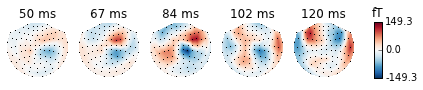In :
fig = evoked.plot_topomap(times=np.linspace(0.05, 0.12, 5), ch_type='grad')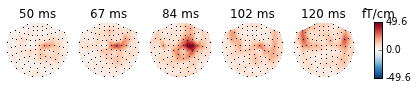In :
evoked.data.shape

Out:
(306, 401)


Save the figure in high resolution pdf

In :
# next line not necessary in Spyder
%matplotlib qt4
import numpy as np
evoked.plot_topomap(times=np.linspace(0.035, 0.1, 5), ch_type='mag', layout=layout)
plt.savefig('som_topo.pdf')
!open som_topo.pdf
# next line not necessary in Spyder
%matplotlib inline


## Exercise:

• Recompute epochs and evoked on highpassed data without baseline. Is it different?

It is also possible to read evoked data stored in a fif file. Let's load the online average provided by the Neuromag system

In :
evoked_fname = data_path + '/meg/somstim_avg.fif'
evoked1 = mne.fiff.read_evoked(evoked_fname, baseline=(None, 0), proj=True)

# fix acquisition
fix_info(evoked1)


Plot it:

In :
fig = evoked1.plot()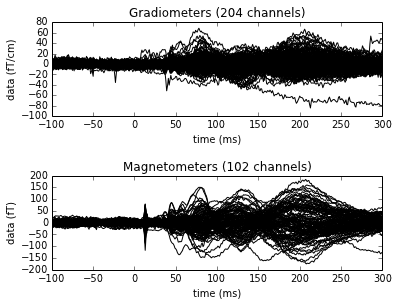## Excercise

• What is the effect of setting proj=True or proj=False in Epochs?

# Summary script

The following cell gives you a full summary script of what's above

In :
import numpy as np
import mne

data_path = '/Users/alex/Sync/karolinska_teaching/'
raw_fname = data_path + '/MEG/somstim_raw.fif'

raw = mne.fiff.Raw(raw_fname)
events = mne.find_events(raw, stim_channel='STI101', verbose=True)

print np.mean(np.diff(events[:, 0]) / raw.info['sfreq'])

#%% fix
def fix_info(raw):
raw.info['chs'][raw.ch_names.index('BIO001')]['kind'] = mne.fiff.constants.FIFF.FIFFV_EOG_CH
raw.info['chs'][raw.ch_names.index('BIO002')]['kind'] = mne.fiff.constants.FIFF.FIFFV_EOG_CH
raw.info['chs'][raw.ch_names.index('BIO003')]['kind'] = mne.fiff.constants.FIFF.FIFFV_ECG_CH
raw.info['chs'][raw.ch_names.index('BIO004')]['kind'] = mne.fiff.constants.FIFF.FIFFV_ECG_CH

fix_info(raw)

#%% compute the Epochs
event_id = dict(som=1)  # event trigger and conditions
tmin = -0.1  # start of each epoch (200ms before the trigger)
tmax = 0.3  # end of each epoch (500ms after the trigger)
baseline = (None, 0)  # means from the first instant to t = 0
reject = dict(grad=4000e-13, mag=4e-12, eog=150e-6)

picks = mne.fiff.pick_types(raw.info, meg=True, eog=True, exclude='bads')
epochs = mne.Epochs(raw, events, event_id, tmin, tmax, proj=True,
picks=picks, baseline=baseline, reject=reject,
epochs.plot_drop_log()

evoked = epochs['som'].average()
evoked.plot()
evoked.plot_topomap(times=np.linspace(0.05, 0.12, 5), ch_type='mag')

Reading 0 ... 825999  =      0.000 ...   825.999 secs...
[done]
400 events found
Events id: 
2.02445112782Out:In []: